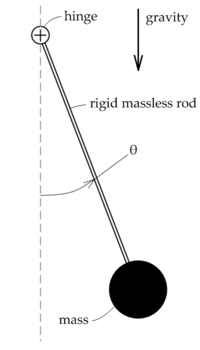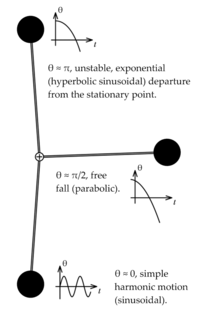# Nonlinear system

Nonlinear system
This article describes the use of the term nonlinearity in mathematics. For other meanings, see nonlinearity (disambiguation).

In mathematics, a nonlinear system is one that does not satisfy the superposition principle, or one whose output is not directly proportional to its input; a linear system fulfills these conditions. In other words, a nonlinear system is any problem where the variable(s) to be solved for cannot be written as a linear combination of independent components. A nonhomogeneous system, which is linear apart from the presence of a function of the independent variables, is nonlinear according to a strict definition, but such systems are usually studied alongside linear systems, because they can be transformed to a linear system of multiple variables.

Nonlinear problems are of interest to engineers, physicists and mathematicians because most physical systems are inherently nonlinear in nature. Nonlinear equations are difficult to solve and give rise to interesting phenomena such as chaos. The weather is famously chaotic, where simple changes in one part of the system produce complex effects throughout.

## Definition

In mathematics, a linear function (or map) f(x) is one which satisfies both of the following properties:

• additivity,$\textstyle f(x + y)\ = f(x)\ + f(y);$
• homogeneity,$\textstyle f(\alpha x)\ = \alpha f(x).$

(Additivity implies homogeneity for any rational α, and, for continuous functions, for any real α. For a complex α, homogeneity does not follow from additivity; for example, an antilinear map is additive but not homogeneous.) The conditions of additivity and homogeneity are often combined in the superposition principle$f(\alpha x + \beta y) = \alpha f(x) + \beta f(y) \,$

An equation written as$f(x) = C\,$

is called linear if f(x) is a linear map (as defined above) and nonlinear otherwise. The equation is called homogeneous if C = 0.

The definition f(x) = C is very general in that x can be any sensible mathematical object (number, vector, function, etc.), and the function f(x) can literally be any mapping, including integration or differentiation with associated constraints (such as boundary values). If f(x) contains differentiation of x, the result will be a differential equation.

## Nonlinear algebraic equations

Nonlinear algebraic equations, which are also called polynomial equations, are defined by equating polynomials to zero. For example,$x^2 + x - 1 = 0\,.$

For a single polynomial equation, root-finding algorithms can be used to find solutions to the equation (i.e., sets of values for the variables that satisfy the equation). However, systems of algebraic equations are more complicated; their study is one motivation for the field of algebraic geometry, a difficult branch of modern mathematics. It is even difficult to decide if a given algebraic system has complex solutions (see Hilbert's Nullstellensatz). Nevertheless, in the case of the systems with a finite number of complex solutions, these systems of polynomial equations are now well understood and efficient methods exist for solving them.

## Nonlinear recurrence relations

A nonlinear recurrence relation defines successive terms of a sequence as a nonlinear function of preceding terms. Examples of nonlinear recurrence relations are the logistic map and the relations that define the various Hofstadter sequences.

## Nonlinear differential equations

A system of differential equations is said to be nonlinear if it is not a linear system. Problems involving nonlinear differential equations are extremely diverse, and methods of solution or analysis are problem dependent. Examples of nonlinear differential equations are the Navier–Stokes equations in fluid dynamics, the Lotka–Volterra equations in biology, and the Black–Scholes PDE in finance.

One of the greatest difficulties of nonlinear problems is that it is not generally possible to combine known solutions into new solutions. In linear problems, for example, a family of linearly independent solutions can be used to construct general solutions through the superposition principle. A good example of this is one-dimensional heat transport with Dirichlet boundary conditions, the solution of which can be written as a time-dependent linear combination of sinusoids of differing frequencies; this makes solutions very flexible. It is often possible to find several very specific solutions to nonlinear equations, however the lack of a superposition principle prevents the construction of new solutions.

### Ordinary differential equations

First order ordinary differential equations are often exactly solvable by separation of variables, especially for autonomous equations. For example, the nonlinear equation$\frac{\operatorname{d} u}{\operatorname{d} x} = -u^2\,$

will easily yield u = (x + C)−1 as a general solution. The equation is nonlinear because it may be written as$\frac{\operatorname{d} u}{\operatorname{d} x} + u^2=0\,$

and the left-hand side of the equation is not a linear function of u and its derivatives. Note that if the u2 term were replaced with u, the problem would be linear (the exponential decay problem).

Second and higher order ordinary differential equations (more generally, systems of nonlinear equations) rarely yield closed form solutions, though implicit solutions and solutions involving nonelementary integrals are encountered.

Common methods for the qualitative analysis of nonlinear ordinary differential equations include:

• Examination of any conserved quantities, especially in Hamiltonian systems.
• Examination of dissipative quantities (see Lyapunov function) analogous to conserved quantities.
• Linearization via Taylor expansion.
• Change of variables into something easier to study.
• Bifurcation theory.
• Perturbation methods (can be applied to algebraic equations too).

### Partial differential equations

The most common basic approach to studying nonlinear partial differential equations is to change the variables (or otherwise transform the problem) so that the resulting problem is simpler (possibly even linear). Sometimes, the equation may be transformed into one or more ordinary differential equations, as seen in the similarity transform or separation of variables, which is always useful whether or not the resulting ordinary differential equation(s) is solvable.

Another common (though less mathematic) tactic, often seen in fluid and heat mechanics, is to use scale analysis to simplify a general, natural equation in a certain specific boundary value problem. For example, the (very) nonlinear Navier-Stokes equations can be simplified into one linear partial differential equation in the case of transient, laminar, one dimensional flow in a circular pipe; the scale analysis provides conditions under which the flow is laminar and one dimensional and also yields the simplified equation.

Other methods include examining the characteristics and using the methods outlined above for ordinary differential equations.

### PendulaIllustration of a pendulumLinearizations of a pendulum

A classic, extensively studied nonlinear problem is the dynamics of a pendulum under influence of gravity. Using Lagrangian mechanics, it may be shown that the motion of a pendulum can be described by the dimensionless nonlinear equation$\frac{d^2 \theta}{d t^2} + \sin(\theta) = 0\,$ (one should note that in this equation g = L = 1)

where gravity points "downwards" and θ is the angle the pendulum forms with its rest position, as shown in the figure at right. One approach to "solving" this equation is to use dθ / dt as an integrating factor, which would eventually yield$\int \frac{d \theta}{\sqrt{C_0 + 2 \cos(\theta)}} = t + C_1\,$

which is an implicit solution involving an elliptic integral. This "solution" generally does not have many uses because most of the nature of the solution is hidden in the nonelementary integral (nonelementary even if C0 = 0).

Another way to approach the problem is to linearize any nonlinearities (the sine function term in this case) at the various points of interest through Taylor expansions. For example, the linearization at θ = 0, called the small angle approximation, is$\frac{d^2 \theta}{d t^2} + \theta = 0\,$

since$\sin(\theta) \approx \theta$ for$\theta \approx 0$. This is a simple harmonic oscillator corresponding to oscillations of the pendulum near the bottom of its path. Another linearization would be at θ = π, corresponding to the pendulum being straight up:$\frac{d^2 \theta}{d t^2} + \pi - \theta = 0\,$

since$\sin(\theta) \approx \pi - \theta$ for$\theta \approx \pi$. The solution to this problem involves hyperbolic sinusoids, and note that unlike the small angle approximation, this approximation is unstable, meaning that | θ | will usually grow without limit, though bounded solutions are possible. This corresponds to the difficulty of balancing a pendulum upright, it is literally an unstable state.

One more interesting linearization is possible around θ = π / 2, around which$\sin(\theta) \approx 1$:$\frac{d^2 \theta}{d t^2} + 1 = 0.$

This corresponds to a free fall problem. A very useful qualitative picture of the pendulum's dynamics may be obtained by piecing together such linearizations, as seen in the figure at right. Other techniques may be used to find (exact) phase portraits and approximate periods.

## Types of nonlinear behaviors

• Indeterminism - the behavior of a system cannot be predicted.
• Multistability - alternating between two or more exclusive states.
• Aperiodic oscillations - functions that do not repeat values after some period (otherwise known as chaotic oscillations or chaos).

## Software for solving nonlinear system

Wikimedia Foundation. 2010.

### Look at other dictionaries:

• nonlinear system — noun a system whose performance cannot be described by equations of the first degree • Hypernyms: ↑system, ↑scheme …   Useful english dictionary

• Nonlinear resonance — In physics, nonlinear resonance is the occurrence of resonance in a nonlinear system. In nonlinear resonance the system behaviour – resonance frequencies and modes – depends on the amplitude of the oscillations, while for linear systems this is… …   Wikipedia

• System identification — In control engineering, the field of system identification uses statistical methods to build mathematical models of dynamical systems from measured data. System identification also includes the optimal design of experiments for efficiently… …   Wikipedia

• Nonlinear autoregressive exogenous model — In time series modeling, a nonlinear autoregressive exogenous model (NARX) is a nonlinear autoregressive model which has exogenous inputs. This means that the model relates the current value of a time series which one would like to explain or… …   Wikipedia

• Nonlinear control — is the area of control engineering specifically involved with systems that are nonlinear, time variant, or both. Many well established analysis and design techniques exist for LTI systems (e.g., root locus, Bode plot, Nyquist criterion, state… …   Wikipedia

• Nonlinear narrative — Nonlinear narrative, disjointed narrative or disrupted narrative is a narrative technique, sometimes used in literature, film, hypertext websites and other narratives, wherein events are portrayed out of chronological order. It is often used to… …   Wikipedia

• Nonlinear Dynamics (journal) — Nonlinear Dynamics, An International Journal of Nonlinear Dynamics and Chaos in Engineering Systems   …   Wikipedia

• system — of forces Earth fixed axis system damped system linear vibrating system force system nonlinear vibrating system statically equivalent force systems tire axis system undamped system vehicle axis system …   Mechanics glossary

• Nonlinear Schrödinger equation — This page is about the equation iψt = −½ψxx + κ|ψ|2ψ. For the equation iψt = −½ψxx + V(x)ψ + κ|ψ|2ψ, used in Bose–Einstein condensate theory, see Gross–Pitaevskii equation. In theoretical physics, the nonlinear Schrödinger equation (NLS) is a… …   Wikipedia

• Nonlinear filter — A nonlinear filter is a signal processing device whose output is not a linear function of its input. Terminology concerning the filtering problem may refer to the time domain (state space) showing of the signal or to the frequency domain… …   Wikipedia